# Developing an Artificial Neural Network in R

In this post, we are going make an artificial neural network (ANN) by analyzing some data about computers. Specifically, we are going to make an ANN to predict the price of computers.We will be using the “ecdat” package and the data set “Computers” from this package. In addition, we are going to use the “neuralnet” package to conduct the ANN analysis. Below is the code for the packages and dataset we are using

``library(Ecdat);library(neuralnet)``
``````#load data set
data("Computers")``````

Explore the Data

The first step is always data exploration. We will first look at nature of the data using the “str” function and then used the “summary” function. Below is the code.

``str(Computers)``
``````## 'data.frame':    6259 obs. of  10 variables:
##  \$ price  : num  1499 1795 1595 1849 3295 ...
##  \$ speed  : num  25 33 25 25 33 66 25 50 50 50 ...
##  \$ hd     : num  80 85 170 170 340 340 170 85 210 210 ...
##  \$ ram    : num  4 2 4 8 16 16 4 2 8 4 ...
##  \$ screen : num  14 14 15 14 14 14 14 14 14 15 ...
##  \$ cd     : Factor w/ 2 levels "no","yes": 1 1 1 1 1 1 2 1 1 1 ...
##  \$ multi  : Factor w/ 2 levels "no","yes": 1 1 1 1 1 1 1 1 1 1 ...
##  \$ premium: Factor w/ 2 levels "no","yes": 2 2 2 1 2 2 2 2 2 2 ...
##  \$ ads    : num  94 94 94 94 94 94 94 94 94 94 ...
##  \$ trend  : num  1 1 1 1 1 1 1 1 1 1 ...``````
``lapply(Computers, summary)``
``````## \$price
##    Min. 1st Qu.  Median    Mean 3rd Qu.    Max.
##     949    1794    2144    2220    2595    5399
##
## \$speed
##    Min. 1st Qu.  Median    Mean 3rd Qu.    Max.
##   25.00   33.00   50.00   52.01   66.00  100.00
##
## \$hd
##    Min. 1st Qu.  Median    Mean 3rd Qu.    Max.
##    80.0   214.0   340.0   416.6   528.0  2100.0
##
## \$ram
##    Min. 1st Qu.  Median    Mean 3rd Qu.    Max.
##   2.000   4.000   8.000   8.287   8.000  32.000
##
## \$screen
##    Min. 1st Qu.  Median    Mean 3rd Qu.    Max.
##   14.00   14.00   14.00   14.61   15.00   17.00
##
## \$cd
##   no  yes
## 3351 2908
##
## \$multi
##   no  yes
## 5386  873
##
##   no  yes
##  612 5647
##
##    Min. 1st Qu.  Median    Mean 3rd Qu.    Max.
##    39.0   162.5   246.0   221.3   275.0   339.0
##
## \$trend
##    Min. 1st Qu.  Median    Mean 3rd Qu.    Max.
##    1.00   10.00   16.00   15.93   21.50   35.00``````

ANN is primarily for numerical data and not categorical or factors. As such, we will remove the factor variables cd, multi, and premium from further analysis. Below are is the code and histograms of the remaining variables.

``hist(Computers\$price);hist(Computers\$speed);hist(Computers\$hd);hist(Computers\$ram)``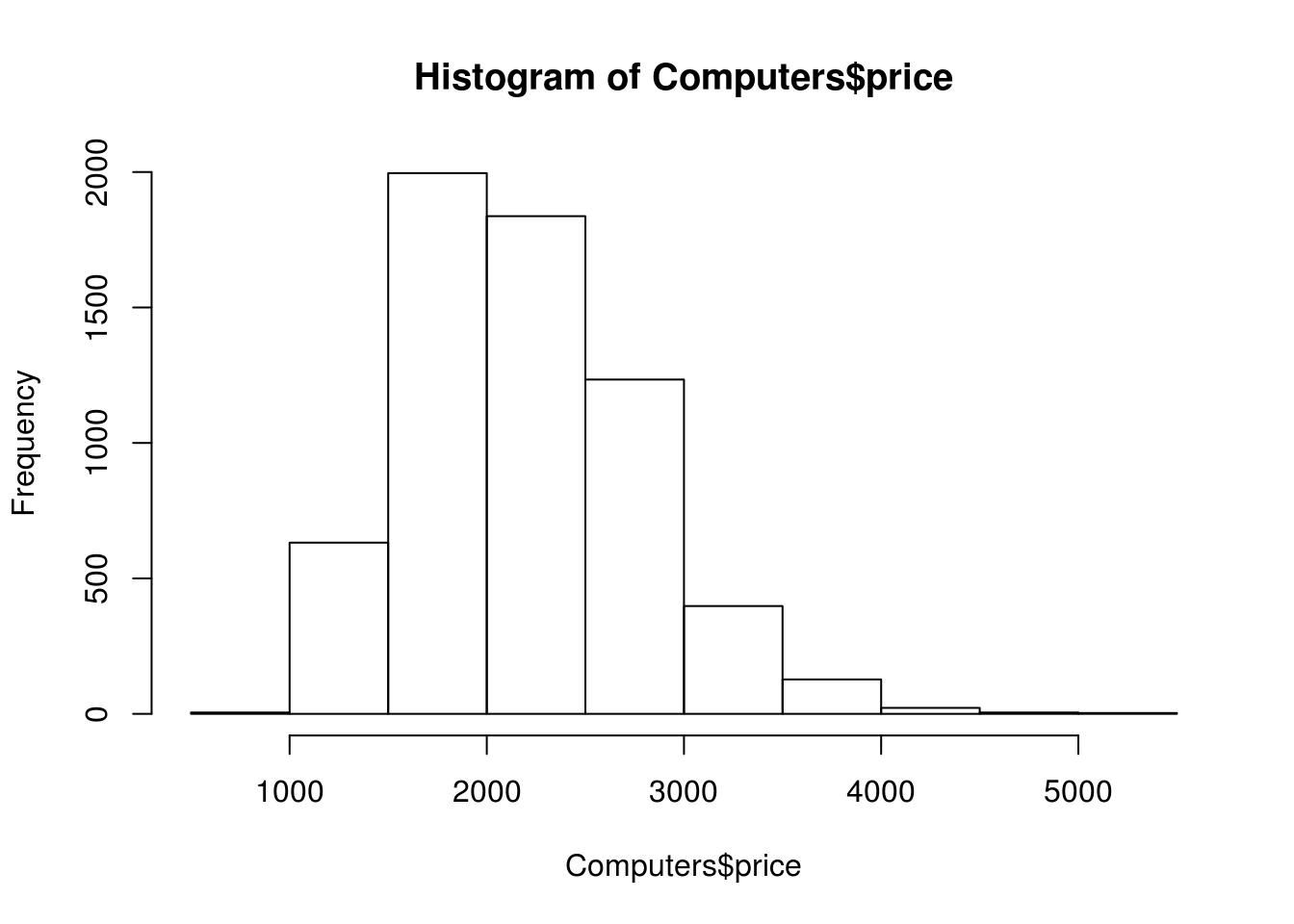``hist(Computers\$screen);hist(Computers\$ads);hist(Computers\$trend)``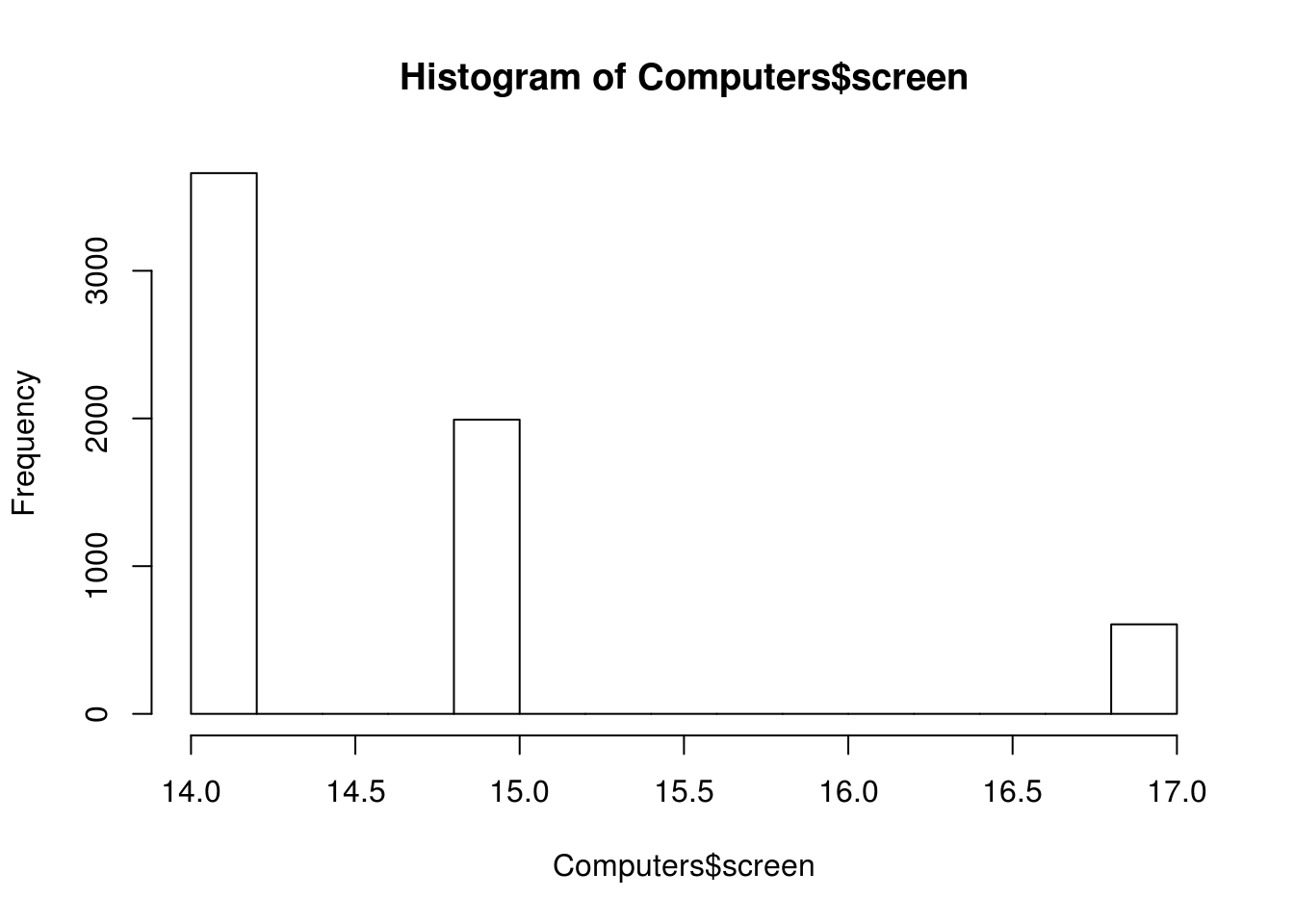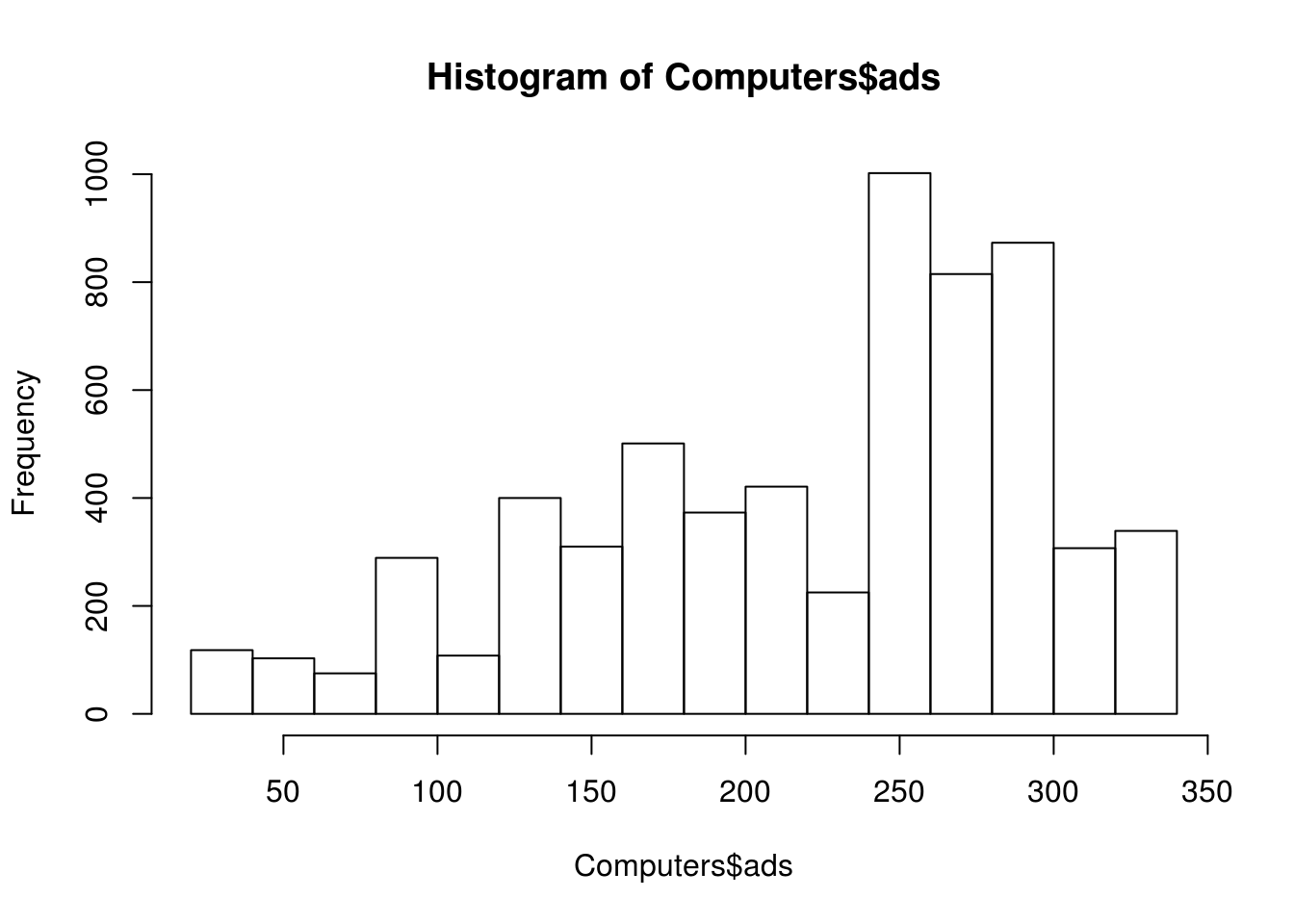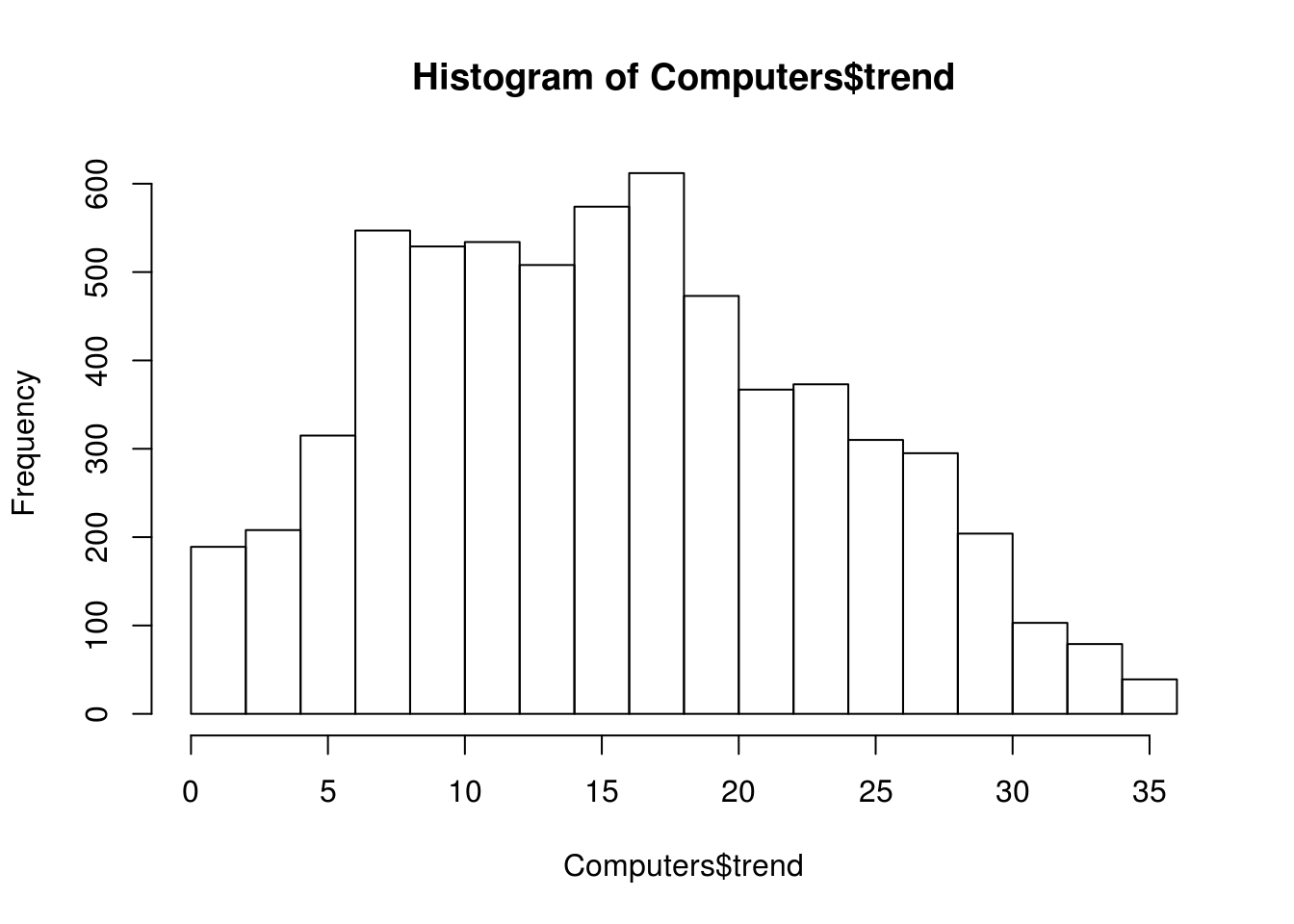Clean the Data

Looking at the summary combined with the histograms indicates that we need to normalize our data as this is a requirement for ANN. We want all of the variables to have an equal influence initially. Below is the code for the function we will use to normalize are variables.

``````normalize<-function(x) {
return((x-min(x)) / (max(x)))
}``````

Explore the Data Again

We now need to make a new dataframe that has only the variables we are going to use for the analysis. Then we will use our “normalize” function to scale and center the variables appropriately. Lastly, we will re-explore the data to make sure it is ok using the “str” “summary” and “hist” functions. Below is the code.

``````#make dataframe without factor variables
Computers_no_factors<-data.frame(Computers\$price,Computers\$speed,Computers\$hd,
Computers\$trend)
#make a normalize dataframe of the data
Computers_norm<-as.data.frame(lapply(Computers_no_factors, normalize))
#reexamine the normalized data
lapply(Computers_norm, summary)``````
``````## \$Computers.price
##    Min. 1st Qu.  Median    Mean 3rd Qu.    Max.
##  0.0000  0.1565  0.2213  0.2353  0.3049  0.8242
##
## \$Computers.speed
##    Min. 1st Qu.  Median    Mean 3rd Qu.    Max.
##  0.0000  0.0800  0.2500  0.2701  0.4100  0.7500
##
## \$Computers.hd
##    Min. 1st Qu.  Median    Mean 3rd Qu.    Max.
## 0.00000 0.06381 0.12380 0.16030 0.21330 0.96190
##
## \$Computers.ram
##    Min. 1st Qu.  Median    Mean 3rd Qu.    Max.
##  0.0000  0.0625  0.1875  0.1965  0.1875  0.9375
##
## \$Computers.screen
##    Min. 1st Qu.  Median    Mean 3rd Qu.    Max.
## 0.00000 0.00000 0.00000 0.03581 0.05882 0.17650
##
##    Min. 1st Qu.  Median    Mean 3rd Qu.    Max.
##  0.0000  0.3643  0.6106  0.5378  0.6962  0.8850
##
## \$Computers.trend
##    Min. 1st Qu.  Median    Mean 3rd Qu.    Max.
##  0.0000  0.2571  0.4286  0.4265  0.5857  0.9714``````
``hist(Computers_norm\$Computers.price);hist(Computers_norm\$Computers.speed);``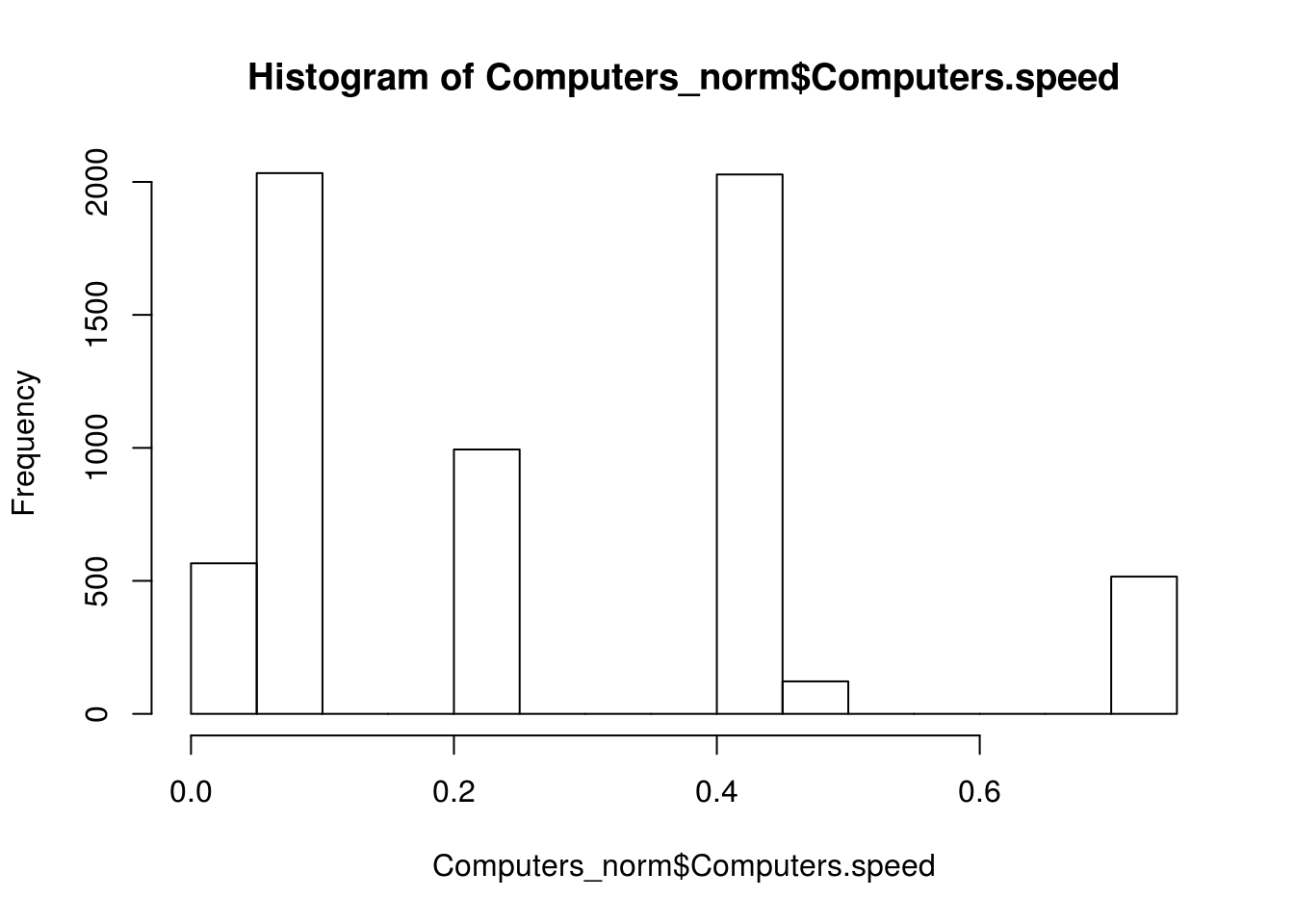``hist(Computers_norm\$Computers.hd);hist(Computers_norm\$Computers.ram);``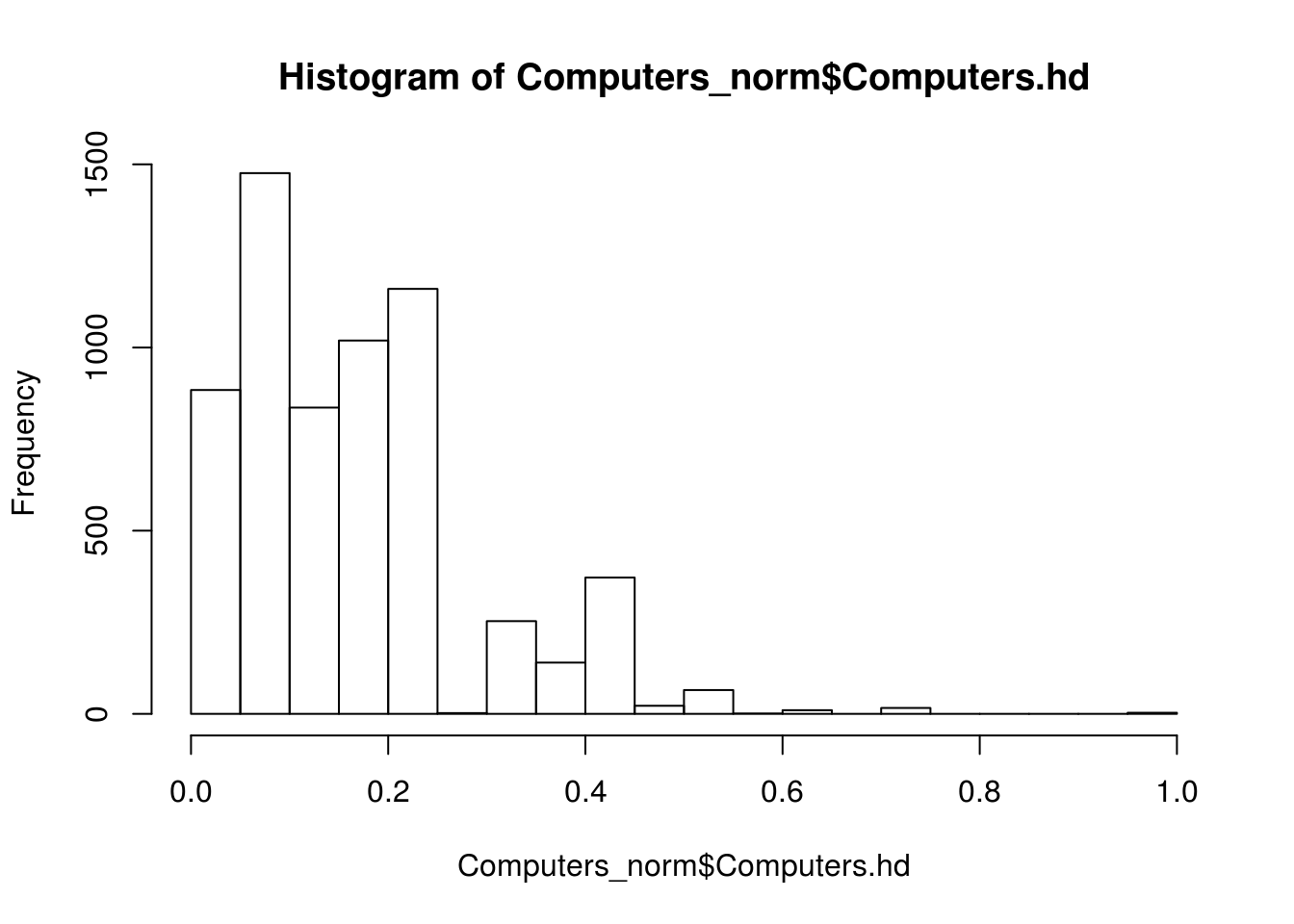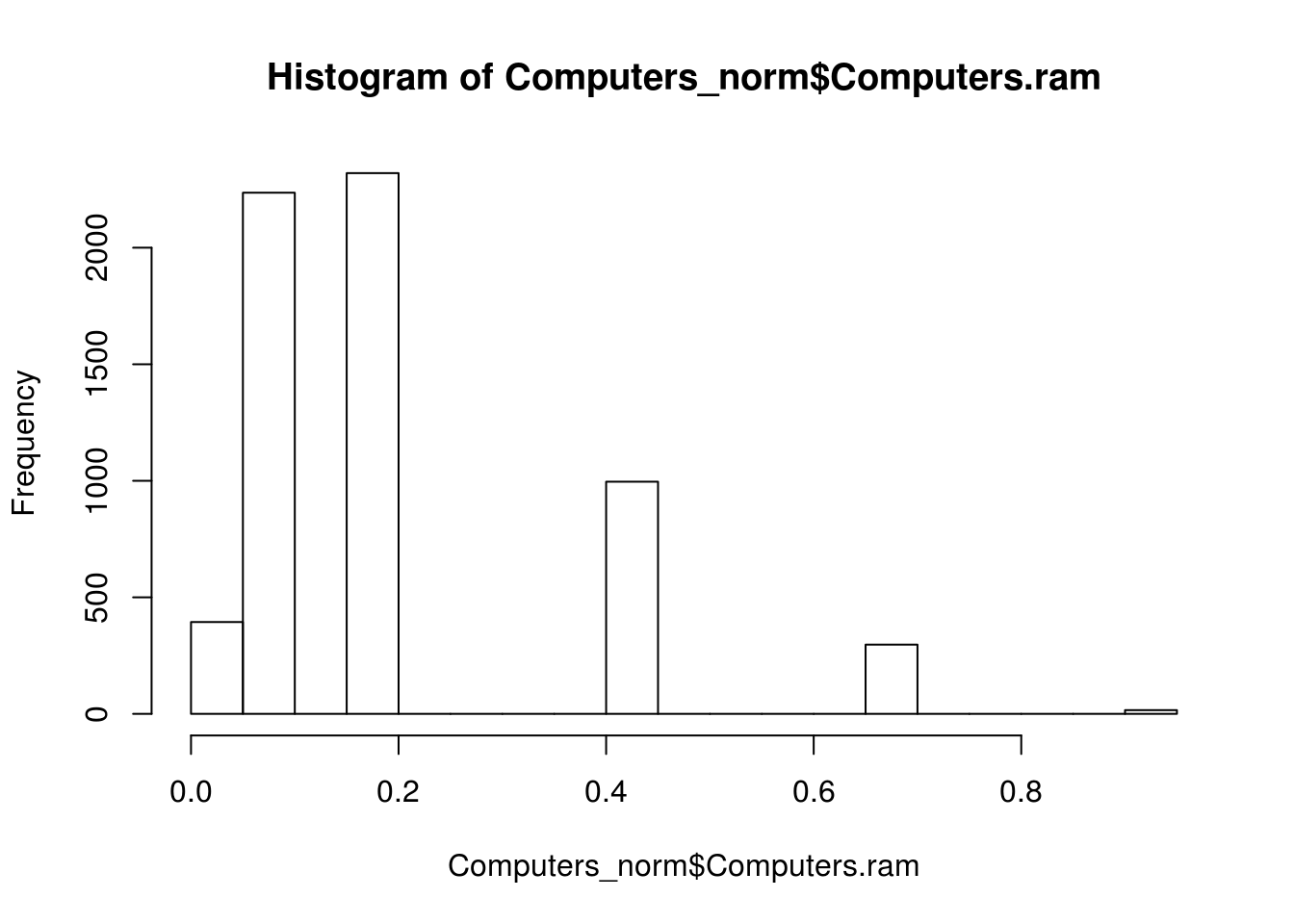``hist(Computers_norm\$Computers.screen);hist(Computers_norm\$Computers.ad);``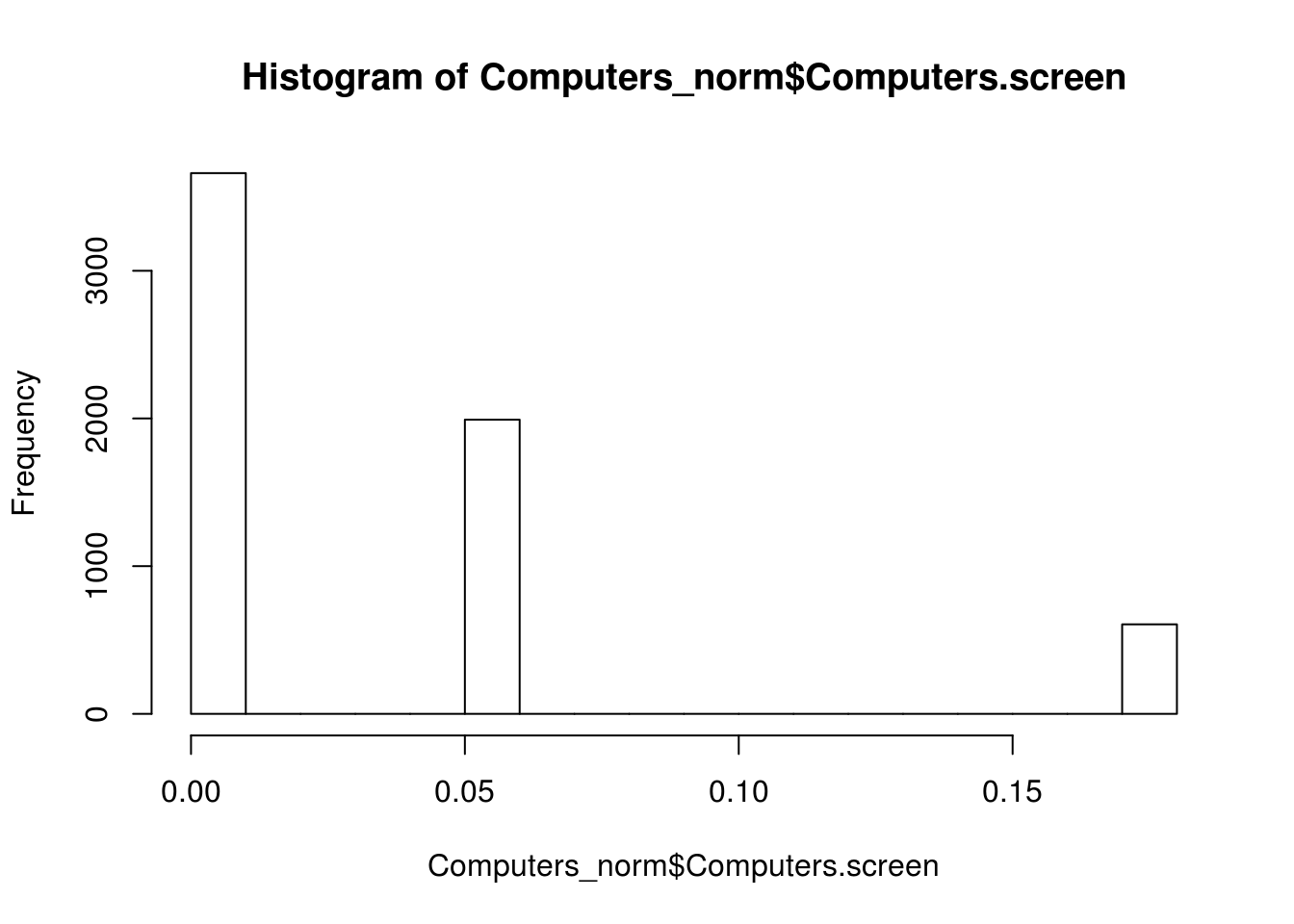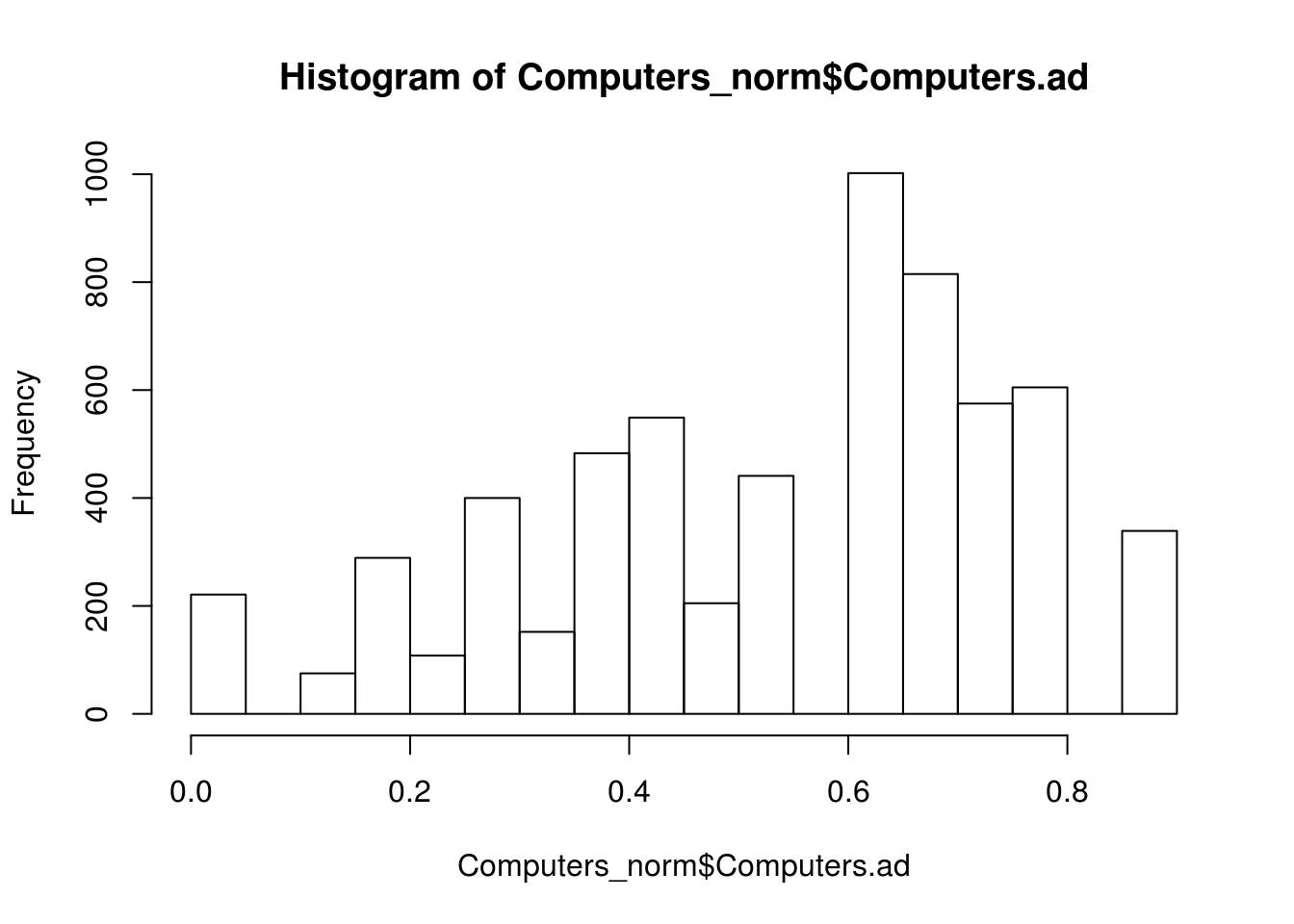``hist(Computers_norm\$Computers.trend)``Develop the Model

Everything looks good, so we will now split our data into a training and testing set and develop the ANN model that will predict computer price. Below is the code for this.

``````#split data into training and testing
Computer_train<-Computers_norm[1:4694,]
Computers_test<-Computers_norm[4695:6259,]
#model
computer_model<-neuralnet(Computers.price~Computers.speed+Computers.hd+
Computers.ram+Computers.screen+

Our initial model is a simple feedforward network with a single hidden node. You can visualize the model using the “plot” function as shown in the code below.

``plot(computer_model)``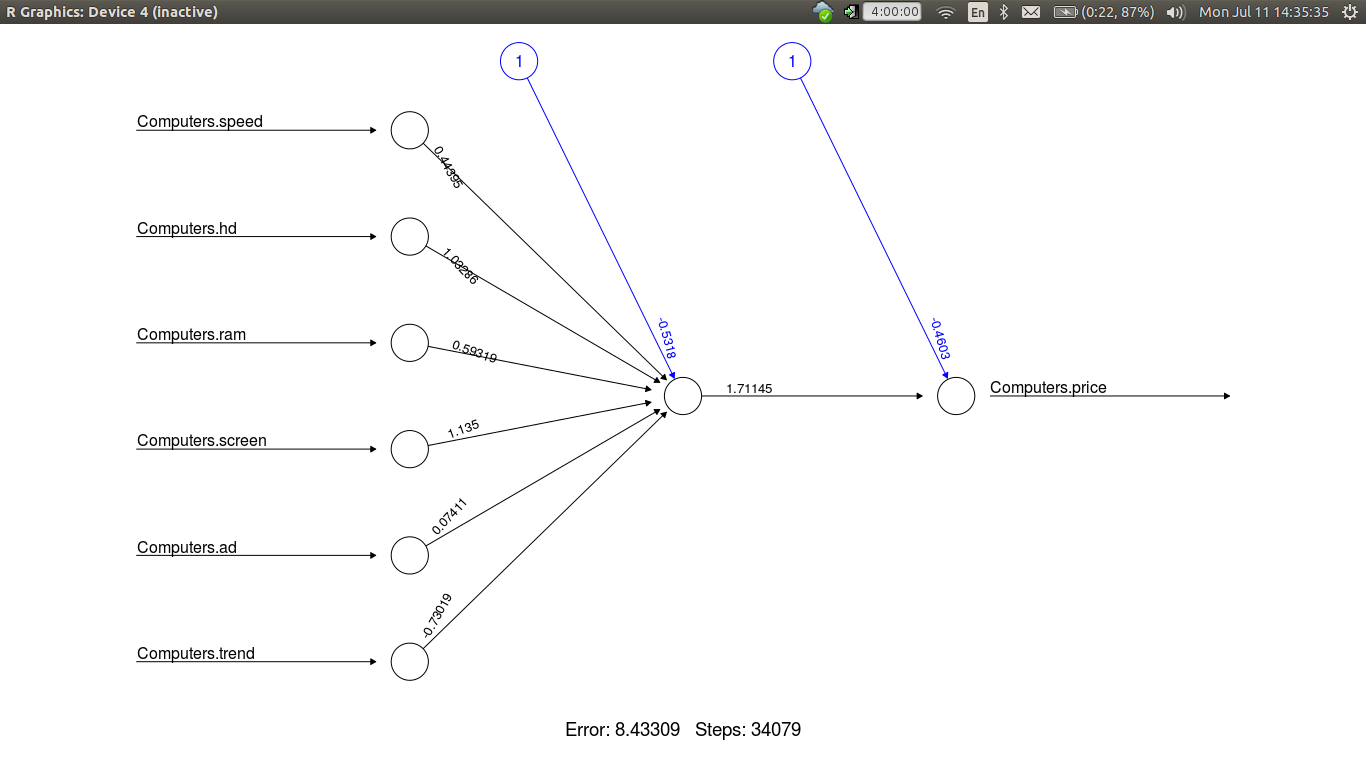We now need to evaluate our model’s performance. We will use the “compute” function to generate predictions. The predictions generated by the “compute” function will then be compared to the actual prices in the test data set using a Pearson correlation. Since we are not classifying we can’t measure accuracy with a confusion matrix but rather with correlation. Below is the code followed by the results

``````evaluate_model<-compute(computer_model, Computers_test[2:7])
predicted_price<-evaluate_model\$net.result
cor(predicted_price, Computers_test\$Computers.price)``````
``````##              [,1]
## [1,] 0.8809571295``````

The correlation between the predict results and the actual results is 0.88 which is a strong relationship. This indicates that our model does an excellent job in predicting the price of a computer based on ram, screen size, speed, hard drive size, advertising, and trends.

Develop Refined Model

Just for fun, we are going to make a more complex model with three hidden nodes and see how the results change below is the code.

``````computer_model2<-neuralnet(Computers.price~Computers.speed+Computers.hd+
Computers.ram+Computers.screen+
Computer_train, hidden =3)
plot(computer_model2)
evaluate_model2<-compute(computer_model2, Computers_test[2:7])
predicted_price2<-evaluate_model2\$net.result
cor(predicted_price2, Computers_test\$Computers.price)
``````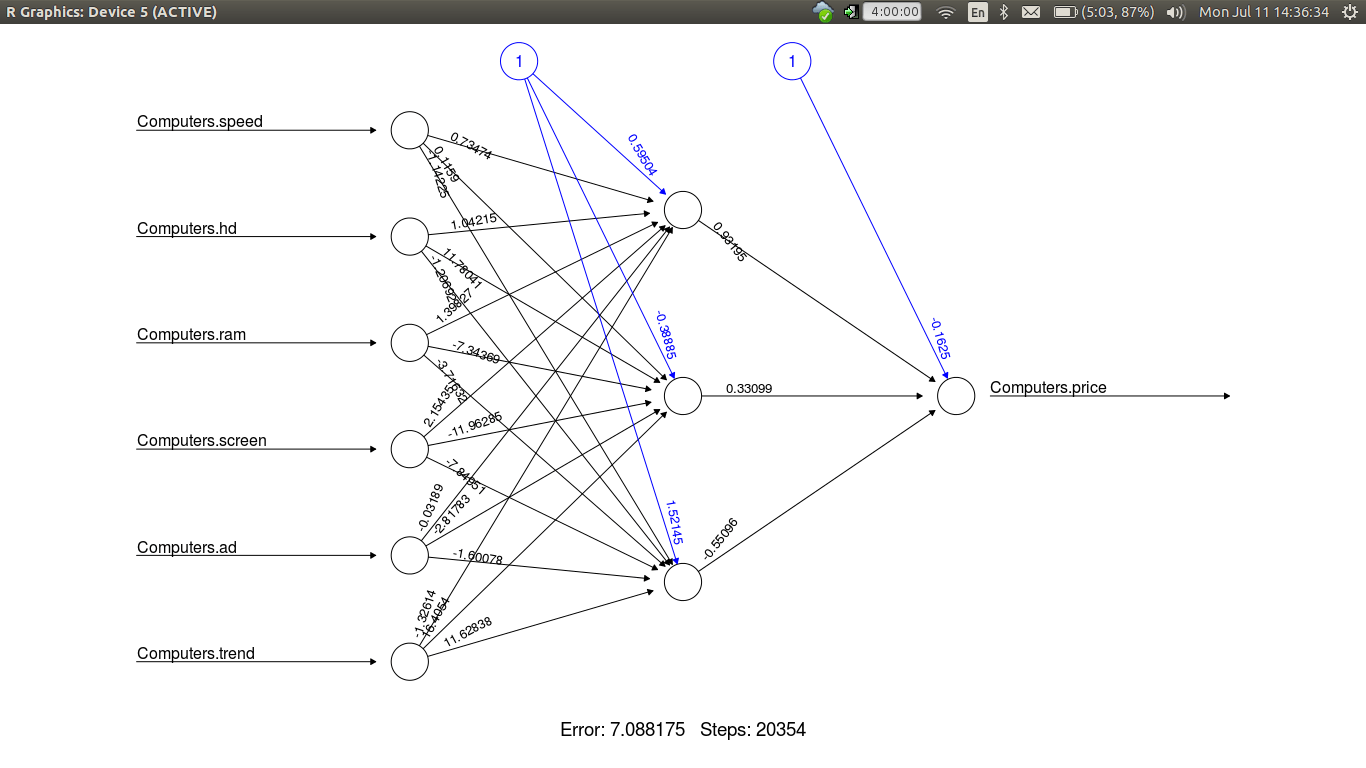``````##              [,1]
## [1,] 0.8963994092``````

The correlation improves to 0.89. As such, the increased complexity did not yield much of an improvement in the overall correlation. Therefore, a single node model is more appropriate.

Conclusion

In this post, we explored an application of artificial neural networks. This black box method is useful for making powerful predictions in highly complex data.

## 3 thoughts on “Developing an Artificial Neural Network in R”

1.The WEDA Coalition

We found this to be brilliant and re-blogged it…thank you